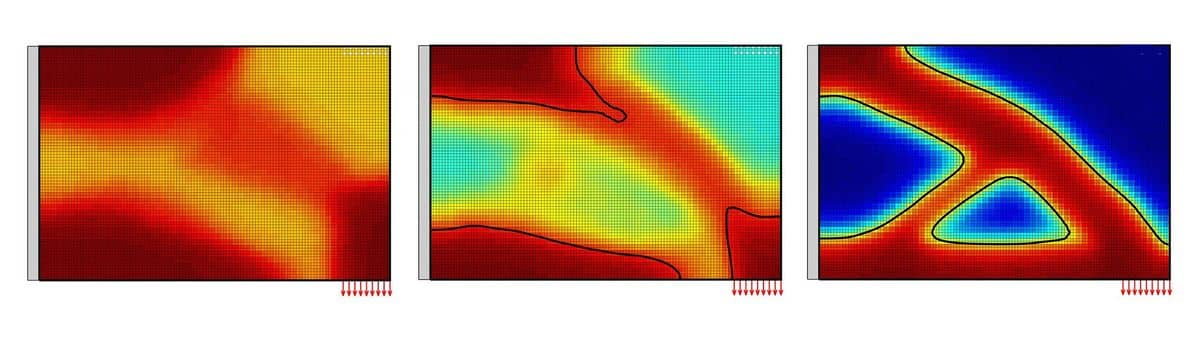# FEATool Topology Optimization Modeling ExampleThis is an advanced model example showing how topology optimization can be implemented and modeled with FEATool. The model has been developed and kindly shared to us by Prof. Shintaro Yamasaki at the Department of Mechanical Engineering of Osaka University .

In contrast to standard simulations where the domain and geometry is known, in topology optimization the problem is reversed and asks what the best shape is for a set of constraints. In the following a material in a plane stress condition fixed to the left wall is being pulled down from the lower right edge, and the question is where to optimally allocate material within the rectangle.To solve this problem the custom PDE equations have been used. One for the plane stress structural mechanics problem, one for the material fraction per cell, and two additional equations for the design variable and node wise filtered material distribution. As this model features very advanced customization and usage it will not be described in further detail but the interested reader can inspect the linked source code.

The images and video below show how the material distribution looks like after a few iterations. One can clearly see how the optimal shape eventually takes on the form of a triangular truss.A MATLAB m-script for the topology optimization model example can be downloaded from the link below. The model depends and requires the `linprog` linear programming solver which is available with both the MATLAB and Octave optimization toolboxes.

FEATool Topology Optimization m-script Model Example

## Reference

 Kawamoto, A., Matsumori, T., Yamasaki, S. et al., Heaviside projection based topology optimization by a PDE-filtered scalar function, Structural and Multidisciplinary Optimization., Volume 44, Issue 1, pp 19-24, Springer-Verlag, 2011.

Category: structural mechanics tutorial

Tags: optimization